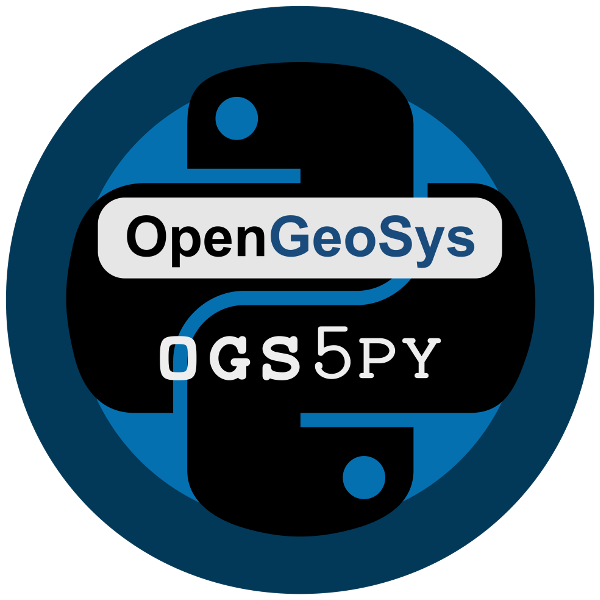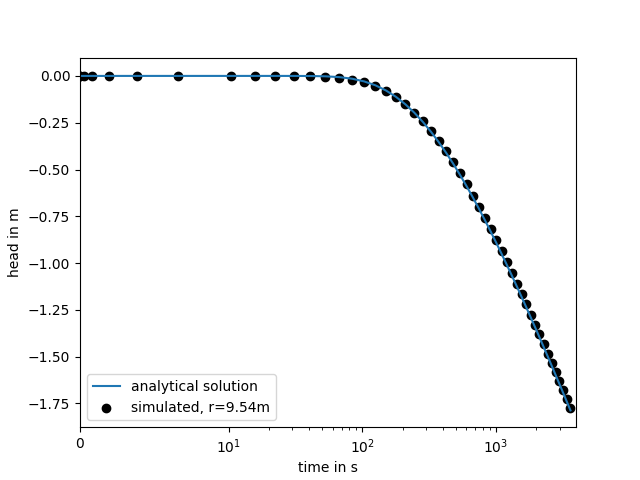# ogs5py Quickstartogs5py is A python-API for the OpenGeoSys 5 scientific modeling package.

## Installation

The package can be installed via pip. On Windows you can install WinPython to get Python and pip running.

```pip install ogs5py
```

Or with conda:

```conda install ogs5py
```

## Citation

If you are using ogs5py in your publication please cite our paper:

Müller, S., Zech, A. and Heße, F.: ogs5py: A Python-API for the OpenGeoSys 5 Scientific Modeling Package. Groundwater, 59: 117-122. https://doi.org/10.1111/gwat.13017, 2021.

You can cite the Zenodo code publication of ogs5py by:

Sebastian Müller. GeoStat-Framework/ogs5py. Zenodo. https://doi.org/10.5281/zenodo.2546767

If you want to cite a specific version, have a look at the Zenodo site.

## Pumping Test Example

In the following a simple transient pumping test is simulated on a radial symmetric mesh. The point output at the observation well is plotted afterwards.

```from ogs5py import OGS, specialrange, generate_time
from matplotlib import pyplot as plt

# discretization and parameters
time = specialrange(0, 3600, 50, typ="cub")
rad = specialrange(0, 1000, 100, typ="cub")
angles = 32
storage = 1e-3
transmissivity = 1e-4
rate = -1e-3
# model setup
# generate a radial mesh and geometry ("boundary" polyline)
PCS_TYPE="GROUNDWATER_FLOW",
GEO_TYPE=["POLYLINE", "boundary"],
DIS_TYPE=["CONSTANT", 0.0],
)
PCS_TYPE="GROUNDWATER_FLOW",
GEO_TYPE=["POINT", "pwell"],
DIS_TYPE=["CONSTANT_NEUMANN", rate],
)
PCS_TYPE="GROUNDWATER_FLOW",
GEO_TYPE="DOMAIN",
DIS_TYPE=["CONSTANT", 0.0],
)
GEOMETRY_DIMENSION=2,
STORAGE=[1, storage],
PERMEABILITY_TENSOR=["ISOTROPIC", transmissivity],
)
PCS_TYPE="GROUNDWATER_FLOW",
LINEAR_SOLVER=[2, 5, 1e-14, 1000, 1.0, 100, 4],
)
PCS_TYPE="GROUNDWATER_FLOW",
GEO_TYPE=["POINT", "owell"],
DAT_TYPE="TECPLOT",
)
model.pcs.add_block(  # set the process type
PCS_TYPE="GROUNDWATER_FLOW", NUM_TYPE="NEW"
)
PCS_TYPE="GROUNDWATER_FLOW",
**generate_time(time)
)
model.write_input()
model.run_model()
```## OGS5 executable

To obtain an OGS5 executable, `ogs5py` brings a download routine `download_ogs`:

```from ogs5py import download_ogs
```

Then a executable is stored in the ogs5py config path and will be called when a model is run.

You can pass a `version` statement to the `download_ogs` routine, to obtain a specific version (5.7, 5.7.1 (win only) and 5.8). For OGS 5.7 there are executables for Windows/Linux and MacOS. For “5.8” there are no MacOS pre-builds.

If you have compiled your own OGS5 version, you can add your executable to the ogs5py config path with `add_exe`:

```from ogs5py import add_exe
```model.run_model(ogs_exe="path/to/ogs")